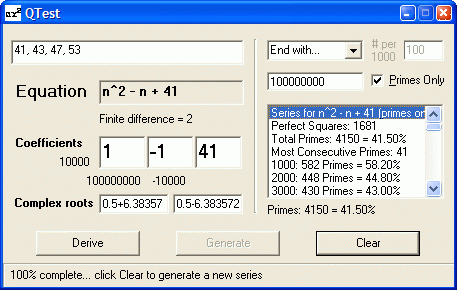NATURAL NUMBERS QTest: Quadratic Testing Toolhome Attention Math Students:     This topic is not related to     the Q test used in statistics. How to Use QTest QTest is a versatile tool for deriving and analyzing quadratic expressions, generating integer sequences, and determining their primality. Download hereDerive To derive a quadratic equation (that is, find its coefficients) and solve* it: Enter 3 or 4 terms and click Derive. Notes: -Use four terms to verify a finite 2nd degree polynomial. -Data can be comma- or space-delimited - or can be a standard list (with line breaks). -You can also enter an equation or coefficients directly. (If you enter an equation directly, click A.) * Although it's not the primary purpose of QTest, solving a quadratic equation means finding its roots - where the parabola of the number sequence intersects the X axis in the Cartesian plane. (Complex roots mean there are no X intersects - therefore, the intersects are imaginary.) Generate Select a range for the generated sequence and other options for generating terms from the expression: -End with* or Start from n =specified number or Random to* or Random from n =specified number. For a random range, select the sampling frequency (between 25 and 975 per 1,000 values of n). -Uncheck Primes only to generate composites also (in which case primes are denoted by a P suffix). -To interrupt, press Esc. (Required for Start from and Random from to stop). *Beginning with n=0. Clear Clears most data, but leaves the quadratic and coefficients, and enables Generate. Shortcut Keys (Press Letter Key Only) Esc = Stop processing, summarizing results so far, and enabling Clear key. Q = Copy sequence (first 10 terms) to Clipboard S = Populate terms box with generated sequence C = Copy complete generated data to Clipboard E = Copy equation to Clipboard A = Populate coefficients from equation Ctrl with Generate = Save data to file in QTest.exe's folder (Generate tooltip shows filename) O with Generate = Shows in parentheses the offset from the pronic sequence (2, 6, 12, 20, 30, 42, ...) N = Copies the selected generated value and the following 3 values into the terms box. Multiple analysis The following steps will generate an analysis of successive quadratic sequences derived from proximate primes, like this: 1. Start and allow caching to finish, then click Derive to enable Generate key. 2. Press P and click Generate to analyze proximate primes up to 1,500, or enter your own range, using a dash, less than 100,000 (for example, 45000-53000). 3. Press G to bulk generate analysis for quadratic expressions. Additional switches: X = Calculate real roots by using absolute discriminants (inverting parabolas with complex roots). D = Include non-quadratic expressions - results for these will not be valid unless linear (for example, 2n + 1) 4. Press C to copy from results window. © 2007 Michael M. Ross